Protocol of a Statistical Evaluation of Some Items in the AD 2006 Code Matrix

An attempt for a statistical analysis of some of the results obtained for the code about AD 2006 has been carried out.

Although it is clear intuitively that the odds for the items in Figures 1 - 4 to be there due to a pure chance are extremely small, some questions have been asked about the estimation of probabilities. These questions are provoked, as far as I am aware, because the items are short words in their majority.

The purpose of this protocol is to demonstrate that the occurrences even of the shortest words have been designed by The Great Encoder. To this end, special attention has been paid not so much to the overall number of occurrences, but to the pattern of the latter.

Statistical data obtained with salvation (ישע) and interpreted

A good example, in my view, is the word salvation (ישע). Consider the matrix shown on Figure 4. It is 51 columns long and 21 rows wide. I decided to leave it 51 columns long in order to illustrate better the contents. It is absolutely symmetrical in relation to the year 2006 (תשרקתשרקו), which occupies a considerable part of the central column. The actual meaningful contents end 1 column short both on the left and the right side. In my view, it is significant that the number of the columns that contain the information appears to be 49 – this is the number of the years between two jubilee years. This fact adds to the idea of timing that the code implies throughout.

However, in order to prevent any suspicions about data manipulation, I decided to examine a number of 51×21 or 51×20 matrices. Matrices have been built as follows. All of them had a central column containing a certain number of letters shin (ש), two of which have always been at a skip interval of 59860 – the skip dividing the two letters in the encoded 2000/6. There are 680 pairs of shin (ש) at this skip in the Torah, so the diversity required for a statistical examination is thus ensured. The other letters shin, if any, were at various places within the column. All matrices were built at skip 14965.

The matrices have been checked for all occurrences of salvation (ישע) at positive skips 2 to 25. The overall number of occurrences of ישע at + 2 to + 25 in the Torah is 1441. The count of the occurrences that are symmetrical in relation to the central column (that is, the shin in the word is among those in the central column) are reported independently.

All matrices have been examined also for salvation (ישע) appearing at any skips entirely within the rectangle formed of the central column and its two adjacent ones. Such occurrence will be called “central” from now on. The central occurrences are also reported in a separate column. The purpose of this examination was to check the odds for random occurrence of the words “winding about the trunk of a tree” shown in Figure 1. Notice that there are three occurrences in accordance with this condition in Figure 1.

Results

Data obtained with matrices containing 3 letters shin (ש) in their central column

Initially, 57 randomly sampled matrices containing exactly 3 letters shin in their central column have been prepared, printed and examined for the items as described above.

The results obtained are presented in the Table and figure below.

Table 1P. Results obtained for salvation (ישע) with 3-ש matrices.

 Number of occurrences of ישע at skips +2 to 25 in a matrix Number of matrices with these occurrences Total number of occurrences in matrices Number of symmetrical occurrences Number of the occurrences in the central 3-column rectangle. 0 6 - - 0 1 17 17 4 *6 2 13 26 0 §4 3 9 27 *4 2 4 9 36 *7 3 5 1 5 0 1 6 2 12 **4 1 Total 57 123 19 17

Note: An asterisk (*) indicates a matrix containing 2 items, while a section sign (§) indicates a matrix containing 3 items of the respective quantities.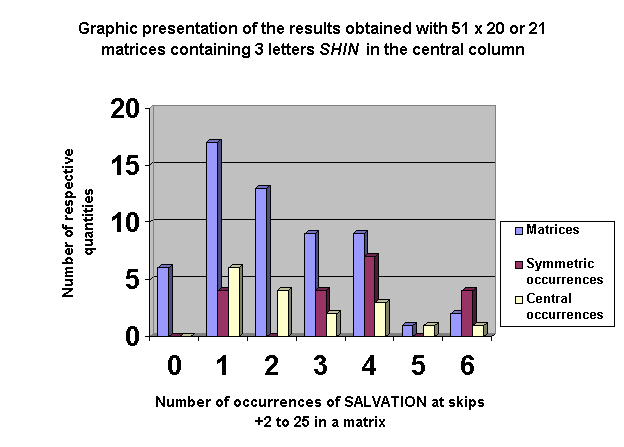Data obtained with matrices containing 2 letters shin (ש) in their central column

In order to confirm these results, matrices containing 2 letters shin and more than 3 letters shin have been prepared. The results obtained are summarized in the tables and figures below.

Table 2P. Results obtained for salvation (ישע) with 2-ש matrices.

 Number of occurrences of ישע at skips +2 to 25 in a matrix Number of matrices with these occurrences Total number of occurrences in matrices Number of symmetrical occurrences Number of the occurrences in the central 3-column rectangle. 0 3 - - *3 1 3 3 1 0 2 7 14 *3 0 3 6 18 0 0 4 4 16 1 0 5 0 - - 0 6 1 6 0 0 Total 24 57 5 3

Note: An asterisk (*) indicates a matrix containing 2 items of the respective quantities.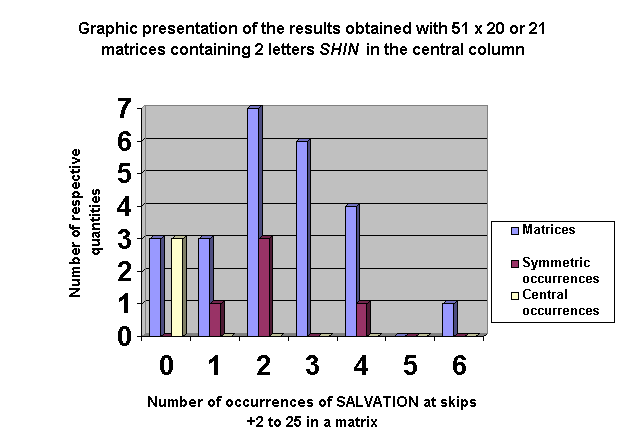Data obtained with matrices containing more than 3 letters shin (ש) in their central column

Table 3P. Results obtained for salvation (ישע) with more than 3-ש matrices.

 Number of occurrences of ישע at skips +2 to 25 in a matrix Number of matrices with these occurrences Total number of occurrences in matrices Number of symmetrical occurrences Number of the occurrences in the central 3-column rectangle. 1 5 5 2 3 2 4 8 0 **¶8 3 3 9 2 1 4 2 8 **4 1 Total 14 30 8 13

Note: An asterisk (*) indicates a matrix containing 2 items, while a paragraph sign (¶) indicates a matrix containing 4 items of the respective quantities.

The distribution of the number of letters shin in the central column of the matrices of this group was as follows:

 4 letters ש 5 letters ש 6 letters ש 7 letters ש 9 3 1 1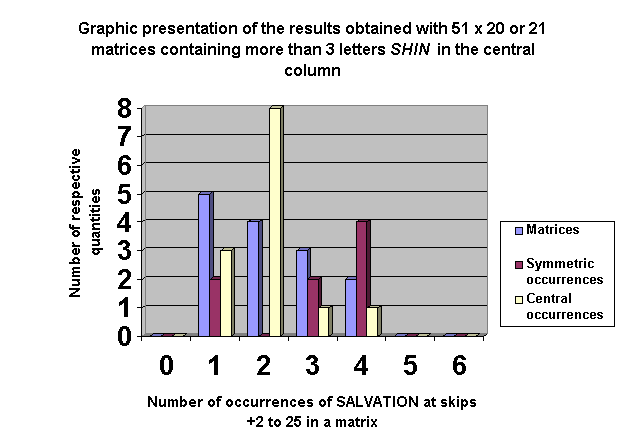Discussion

The average number of occurrences of the three-letter word salvation (ישע) in Hebrew appeared to be relatively constant, independent on the number of the letters shin in the central column:

2 letters shin in the central column (Table 2P): 57/24 = 2.38 occurrences per matrix;

3 letters shin in the central column (Table 1P): 123/57 = 2.16 occurrences per matrix;

>3 letters shin in the central column (Table 3P): 30/14 = 2.14 occurrences per matrix.

On the other hand, the occurrences in the central 3-column rectangle show a high dependence on the number of shins in the central column:

2 letters shin in the central column (Table 2P): 3/24 = 0.125 occurrences per matrix;

3 letters shin in the central column (Table 1P): 17/57 = 0.298 occurrences per matrix;

>3 letters shin in the central column (Table 3P): 13/14 = 0.929 occurrences per matrix.

These results could be expected – the higher number of shins leads to higher probabilities for occurrence of a word with a shin in its centre. The same is valid for the rate of symmetrical occurrences:

2 letters shin in the central column (Table 2P): 5/24 = 0.208 occurrences per matrix;

3 letters shin in the central column (Table 1P): 19/57 = 0.333 occurrences per matrix;

>3 letters shin in the central column (Table 3P): 8/14 = 0.571 occurrences per matrix.

It is evident from the above data that the rate of occurrence of both symmetrical and central ישע in all three cases is lower than 1. This is especially valid in the cases of 2- and 3-letter shin in the central column. Therefore, it seems that the most appropriate method for evaluation of the probabilities for symmetrical and central occurrences is the Poisson Distribution.

Poisson distribution is described by the general formula

λn

P(n) = ——— e-λ

n!

P(n) is the probability for n items to be found in a matrix if the probability follows the Poisson distribution. λ is called distribution parameter and it corresponds to the overall number of occurrences of a quantity spread among the total number of matrices. e is the base of the natural logarithms.

In order to use this formula for the estimation of probabilities for n = 4 (symmetrical occurrences) and n = 3 (central occurrences) in first place we must check whether the data obtained agree with the formula.

According to the Poisson distribution, the expected number of matrices that do not contain an item (that is, n = 0) will be e-λ; the expected number of those containing one item will be λe-λ, etc.

Let us explore the data obtained for symmetrical appearances in the matrices containing 3 letters shin in their central column. λ, as calculated above, is 19/57 = 0.3333. It is evident from the fourth column (coloured in plum) in Table 1P that the number of matrices with two symmetrical occurrences of salvation (ישע) is 4. Hence the number of matrices with one occurrence is 19 – 4×2 = 11. The number of those, which do not contain this item, is 42. The results are summarized in Table 4P. Deviation in terms of (Expected – Observed)2/Expected is given for the purpose of calculation the chi-squared (χ2) criterion.

Table 4P. Expected values according to Poisson distribution and actual data obtained with matrices with 3 letters shin (ש) in their central column containing symmetrical occurrences of salvation (ישע) at skips +2 to 25.

 Number of occurrences in a matrix Expected Observed (O) Deviation Proportion Number (E) O - E (O – E)2/E 0 0.7165 40.8 42 +1.2 0.03529 1 0.2388 13.6 11 -2.6 0.49706 2 0.0399 2.3 4 +1.7 1.25652

The calculations with the central occurrences in the matrices containing 3 letters shin in their central column are summarized in Table 5P.

Table 5P. Expected values according to Poisson distribution and actual data obtained with matrices with 3 letters shin (ש) in their central column containing central occurrences of salvation (ישע) at any skips. λ = 17/57 = 0.2982.

 Number of occurrences in a matrix Expected Observed (O) Deviation Proportion Number (E) O - E (O – E)2/E 0 0.7422 42.3 43 +0.7 0.01158 1 0.2213 12.6 12 -0.6 0.02857 2 0.0330 1.9 1 -0.1 0.00476 3 0.0033 0.2 1

The same type of calculations for the matrices containing 2 letters shin in their central column are presented in Tables 6P and 7P.

Table 6P. Expected values according to Poisson distribution and actual data obtained with matrices with 2 letters shin (ש) in their central column containing symmetrical occurrences of salvation (ישע) at skips +2 to 25. λ = 5/24 = 0.2083.

 Number of occurrences in a matrix Expected Observed (O) Deviation Proportion Number (E) O - E (O – E)2/E 0 0.8119 19.5 20 +0.5 0.01282 1 0.1691 4.1 3 -1.1 0.29512 2 0.0176 0.4 1 +0.6 0.90000

Table 7P. Expected values according to Poisson distribution and actual data obtained with matrices with 2 letters shin (ש) in their central column containing central occurrences of salvation (ישע) at any skips. λ = 3/24 = 0.1250.

 Number of occurrences in a matrix Expected Observed (O) Deviation Proportion Number (E) O - E (O – E)2/E 0 0.8825 21.2 22 +0.8 0.03019 1 0.1103 2.6 1 -0.8 0.22857 2 0.0069 0.2 1

These calculations for the matrices containing more than 3 letters shin in their central column produced the results presented in Tables 8P and 9P.

Table 8P. Expected values according to Poisson distribution and actual data obtained with matrices with >3 letters shin (ש) in their central column containing symmetrical occurrences of salvation (ישע) at skips +2 to 25. λ = 8/14 = 0.5714.

 Number of occurrences in a matrix Expected Observed (O) Deviation Proportion Number (E) O - E (O – E)2/E 0 0.5647 7.9 8 +0.1 0.00127 1 0.3227 4.5 4 -0.5 0.05556 2 0.0922 1.3 0 -1.3 1.30000

Table 9P. Expected values according to Poisson distribution and actual data obtained with matrices with >3 letters shin (ש) in their central column containing central occurrences of salvation (ישע) at any skips. λ = 13/14 = 0.9286.

 Number of occurrences in a matrix Expected Observed (O) Deviation Proportion Number (E) O - E (O – E)2/E 0 0.3950 5.5 6 +0.5 0.04545 1 0.3668 5.1 5 -0.1 0.00196 2 0.1703 2.4 2 -0.4 0.06667 3 0.0527 0.7 0 +0.1 0.01111 4 0.0122 0.2 1

Conclusion about the Suitability of the Method

The criterion most widely used for the verification of a hypothesis for a distribution pattern is the chi-squared value. Let us check the results using this criterion. The deviations shown in the last two columns in the tables have been corrected for the classes with expected items below 0.4. Such corrections are recommended for the bordering classes in case the expected number E is < 1. The recommendation in such cases is to enlarge the class combining it with the neighbouring one. In our cases, these values were combined with the previous class and hence the observed values have been summed up in the same way. Then, aggregate values for the deviation have been calculated. Chi-squared value is calculated according to the formula

(Observed – Expected)2

χ2 = ——————————

Expected

Summing is performed for all classes in a group.

The χ2 values calculated are summarized in the following table.

 χ2 for symmetric occurrences χ2 for central occurrences 2-ש matrices 1.21 0.26 3- ש matrices 1.79 0.04 >3-ש matrices 1.37 0.13

It is obvious from the figures in the table that the Poisson distribution hypothesis is confirmed. On the other hand, no dependence between the number of symmetric and central occurrences in a matrix can be inferred from Tables 1P – 3P and the graphic presentations below them. Also, definitely no dependency could be derived between the number of central occurrences and the overall number of occurrences at skips +2 to 25 in a matrix. On the other hand, it is quite naturally that the higher is the number of the latter, the higher should be the probability for one of them to be a symmetric one. But we have constructed our proposition on the basis of preliminarily unknown overall number of occurrences at skips +2 to 25 in the matrix observed.

Therefore, the Poisson distribution can be applied for the calculation of the probabilities for symmetrical and central occurrences of the 3-letter word salvation (ישע) in the 51×21 matrix shown on Figure 4 and then the probability for the concurrent event.

Calculation of probability

Note: Because the probabilities are approximate, in all calculations of their values, the differences between matrices containing 20 and 21 rows have been ignored. The chances for 21 rows are of the order of 1.03, or just 3%, higher than the mean value.

The probability for 4 random symmetric occurrences of salvation (ישע) at positive skips 2 to 25 in a 51×20 or 21 matrix is

Ps(4) = (0.3333^4/4!)×e^-0.3333 = 3.685×10^-4

or Ps(4) = 1/2,714.

The probability for 3 random central occurrences of salvation (ישע) at any skips in a 51×20 or 21 matrix is

Pc(3) = (0.2982^3/3!) ×e^-0.2982 = 0.00328

or Pc(3) = 1/305.

Hence the overall probability for the random occurrences of the items salvation (ישע) complying with the requirements according to the test is

Ps(4) × Pc(3) = 3.685×10^-4 × 0.00328 = 1.209×10^-6

or P = 1/827,000.

But a biased and skeptic one may claim that we haven’t specified positive skips for the symmetric occurrences. Let us check the probability in this case.

The overall number of occurrences of ישע at - 2 to - 25 in the Torah is 1470. This is only 2% higher than 1441 - the number of the occurrences at the respective positive skip. Therefore, we can reasonably expect a doubled rate of occurrences, or λ = 0.3333×2 = 0.6667. In this case, however, the number of occurrences is already five. Thus the probability for 5 random symmetric occurrences of salvation (ישע) at skips ±2 to 25 in a 51×20 or 21 matrix should be

Ps(5) = (0.6667^5/5!)e^-0.6667 = 5.635×10^-4

or Ps(5) = 1/1,775.

In this case, the overall probability for the random occurrences of the items salvation (ישע) complying with the requirements according to the test is

Ps(5) × Pc(3) = 5.635×10^-4 × 0.00328 = 1.848×10^-6

or P = 1/541,000.

As it appears, even a skeptical approach to the evaluation of the probability will lead to a value of the order of one in a half million.

Interpretation of the Rate of Appearances of Heaven (השמים) in the Plain Text and the Encoded Occurrence

Another good example in my view is the word heaven (השמים). The estimation of the rate of appearance in the plain text is valid because the only passage in the whole Torah where God explicitly says “I have spoken from heaven” is in the matrix.

I tried to expose this fact in the matrix shown on Figure 3. However, there are shown only four occurrences because I retained the central place for the column containing the year 2006 (תשרקתשרקו) in order to emphasize its importance. In fact, a matrix with a lower numbers of columns could be constructed (see Figure 5), containing five appearances of heaven (red ovals) in the plain text as well as the encoded word (blue ovals) at skip, which, remarkably, is 5. The year 2006 is in black ovals. The matrix is 67 columns long. Let us estimate the probability for finding at random 5 words השמים in such a matrix.

Heaven (השמים) appears 81 times in the plain text of the Torah. The whole text of the Torah is about 223 times 67-columns long matrices at skip 14965 (containing either 20 or 21 rows). If the 81 words השמים are considered randomly spread, the probability for finding one of them in a matrix of the specified parameters will be 81/223 = 0.3626. In fact, they are not evenly spread: 13 of them (16 %) are within the first 2650 letters of the text. Also, there are several pairs of these words (less than 10 pairs) that are at distances below 100 along the text. Two of these pairs are found in Figure 5. If we accept the approach of finding pairs, however, the calculated probability will prove to be even lower compared to the random-spread approach.

Because we know the likelihood for a random appearance of heaven in the matrix, the simplest way of estimation the probability for finding five words in the matrix is to raise this known value to the power of 5:

P(5) = 0.3626^5 = 0.006268

or P(5) = 1/160

There is also the encoded word heaven at skip 5 in the matrix. There are 7 occurrences of heaven (השמים) at skips ±2 to 5 in the Torah. Therefore, the probability for finding one of them in the matrix will be merely

P(H) = 7/223 = 0.03139

or P(H) = 1/31.9

Hence the overall probability for a random occurrence of five words in the plain text and an encoded word at skip ±2 to 5 appears to be

P(5) × P(H) = 0,006268×0.03139= 0.0001967

or PHeaven = 1/5,080

Another interesting matrix I managed to construct while preparing this document is the absolutely symmetrical one shown in Figure 6. The designations are the same as in Figure 5. The matrix is of skip 14964 and contains 63 columns. There are four words heaven in the plain text plus the encoded word. The amazing fact is that the number of letters in ovals to the left and to the right of the geometrical centre is the same: 16. There are two letters in the central column: tav (ת) and mem (מ). They form the two-letter word תם at skip -104748. This is what is written for two words of the same spelling in the Wilson’s Old Testament Word Studies :

ם(tam) perfect, plain, undefiled, upright;

ם(tom) full, integrity, perfect, perfection, simplicity, Thummim, upright, uprightly, uprightness.

Although a short one, this word fits rightly in its place. The meaning is in full harmony with the idea of conclusion and perfection that emanates from this strange code.

The perfection is underlined by the symmetric vertical positioning of the השמים that gives the mem to form the 2-letter word and the encoded one in the bottom row.

But there is more in the central column. Already a 3-letter word is formed there including these two letters, but at skip +104748:

תמ (matat) gift, to give, reward .

General Conclusion on Probabilities

The probability for the concomitant finding of the items discussed in this paper can be calculated as the product of the probabilities at each stage. Thus, even if we confine to the matrix in Figure 4, where are two of the words heaven at skip +1 wholly contained in the matrix and the encoded one at skip 5 and the findings about salvation, and carry out the adjustments required for the passing from a 67-column matrix to a 51-column one, yet a skeptical evaluation will produce a probability as low as

P = 1.848×10^-6×0.2760^2×0.02385 = 3.358×10^-9

or P = 1/300,000,000.

The combined probability for salvation and heaven appears to be one in three hundred millions! And yet many of the crucial words found in the matrix haven’t entered so far!

Lyuben Piperov

July 15th 2004

Sofia, Bulgaria

Contact Lyuben Piperov at l_piperov@yahoo.co.uk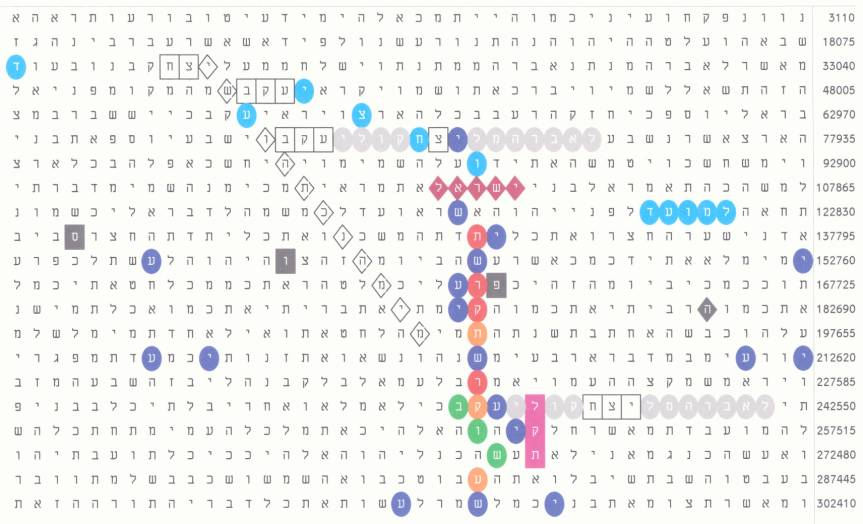Figure 1.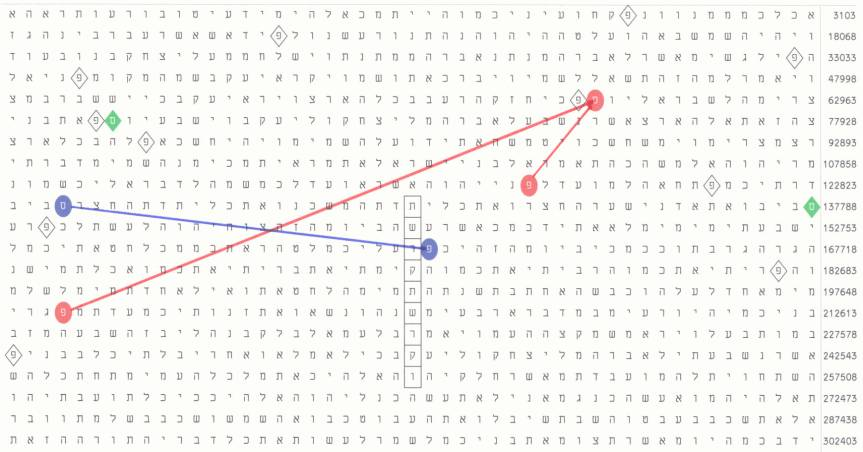Figure 2.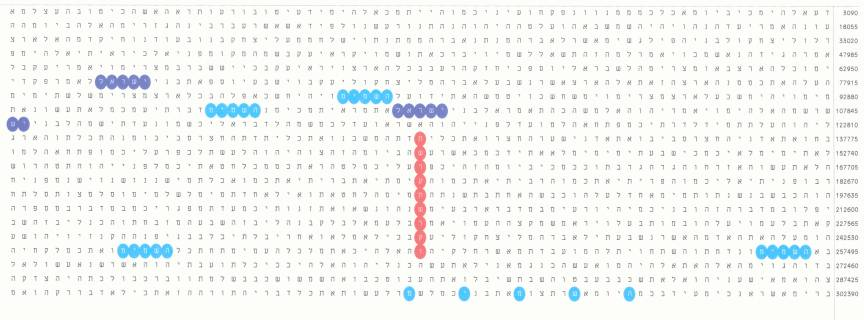Figure 3.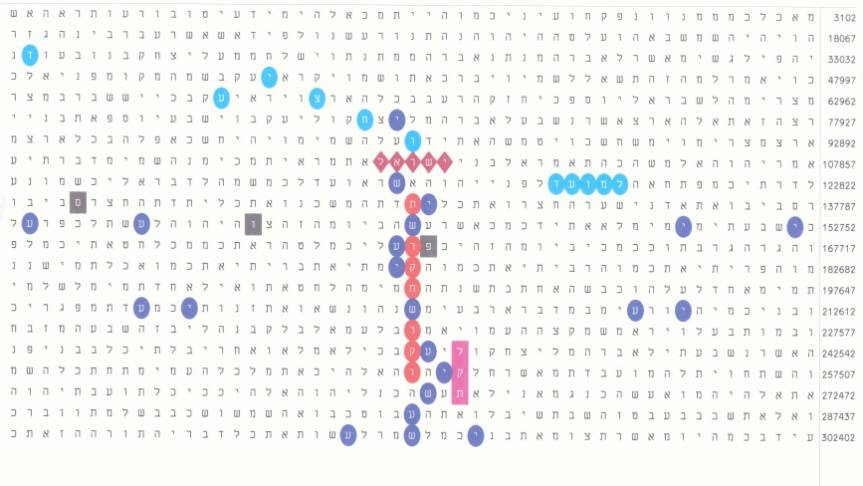Figure 4.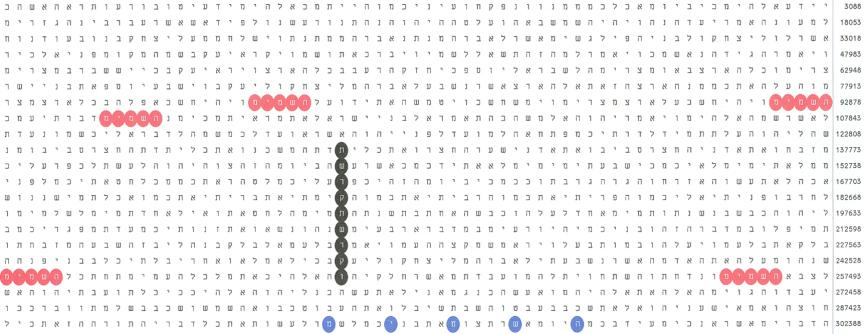Figure 5Figure 6